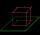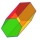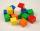# Pythagorean theorem + area - math problems

#### Number of problems found: 353

• Tetrahedral pyramidWhat is the surface of a regular tetrahedral (four-sided) pyramid if the base edge a=16 and height v=16?
• 3s prismIt is given a regular perpendicular triangular prism with a height of 19.0 cm and a base edge of 7.1 cm. Calculate the volume of the prism.
• How toHow to find a total surface of a rectangular pyramid if each face is to be 8 dm high and the base is 10 dm by 6 dm.
• Pyramid heightFind the volume of a regular triangular pyramid with edge length a = 12cm and pyramid height h = 20cm.
• Cube cutThe cube ABCDA'B'C'D ' has an edge of 12cm. Calculate the area of diagonal cut B DD'B '.
• HexagonCalculate the regular hexagonal prism's surface whose base edge a = 12cm and side edge b = 3 dm.
• The volumeThe volume of the cone is 94.2 dm³, the radius of the base is 6 dm. Calculate the surface of the cone.
• Sphere cutsAt what distance from the center intersects sphere with radius R = 56 plane, if the cut area and area of the main sphere circle is in ratio 1/2.
• Above EarthTo what height must a boy be raised above the earth to see one-fifth of its surface.
• PilotHow high is the airplane's pilot to see 0.001 of Earth's surface?
• Cube - wallV kocke ABCDEFGH je |CF|=4 √ 2. Aký je povrch kocky?
• AirplaneAviator sees part of the earth's surface with an area of 200,000 square kilometers. How high he flies?
• Solid cuboidA solid cuboid has a volume of 40 cm3. The cuboid has a total surface area of 100 cm squared. One edge of the cuboid has a length of 2 cm. Find the length of a diagonal of the cuboid. Give your answer correct to 3 sig. Fig.

We apologize, but in this category are not a lot of examples.
Do you have an exciting math question or word problem that you can't solve? Ask a question or post a math problem, and we can try to solve it.

We will send a solution to your e-mail address. Solved examples are also published here. Please enter the e-mail correctly and check whether you don't have a full mailbox.

Pythagorean theorem is the base for the right triangle calculator. Pythagorean theorem - math word problems. Area - math word problems.